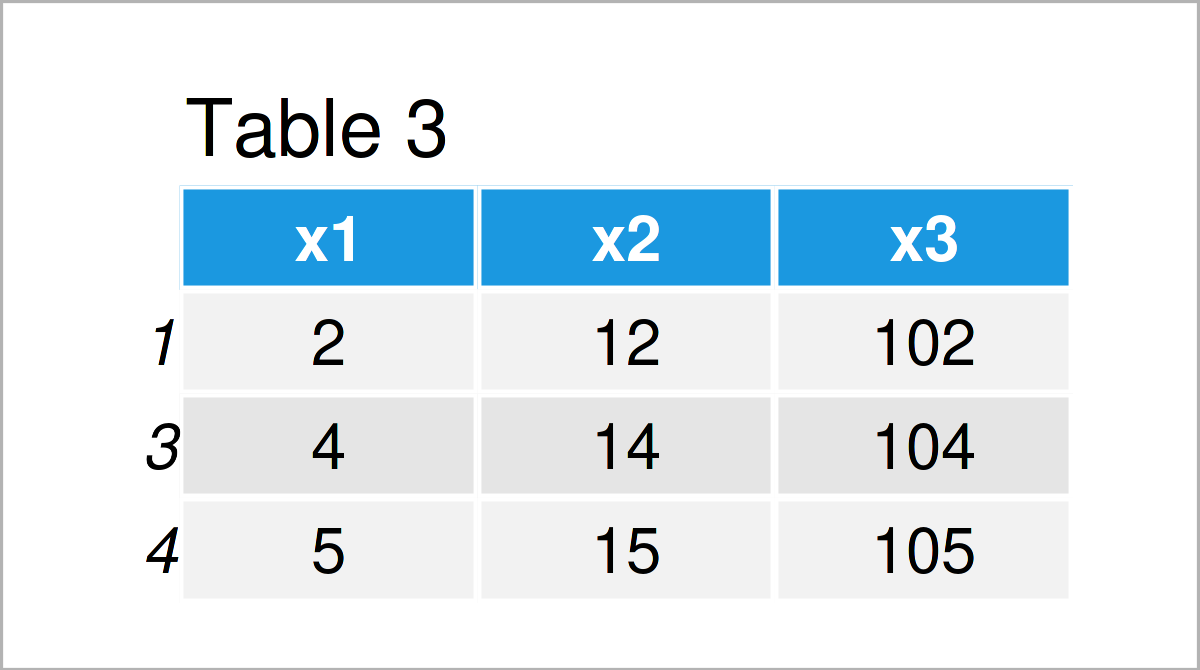# Select Rows of pandas DataFrame by Index in Python (2 Examples)

In this Python tutorial you’ll learn how to extract pandas DataFrame rows by index positions.

The tutorial contains these contents:

You’re here for the answer, so let’s get straight to the examples!

## Example Data & Software Libraries

To be able to use the functions of the pandas library, we first have to load pandas:

`import pandas as pd # Load pandas`

Let’s also create some exemplifying data.

```data = pd.DataFrame({'x1':range(1, 6), # Create example DataFrame 'x2':range(11, 16), 'x3':range(101, 106)}) print(data) # Print example DataFrame```Table 1 visualizes the output of the Python console that has been returned after running the previous Python programming code and shows that our example data is constructed of five rows and three columns called “x1”, “x2”, and “x3”.

## Example 1: Extract Single Row from pandas DataFrame by Index

In this example, I’ll demonstrate how to return exactly one line of a pandas DataFrame.

For this, we can use the iloc attribute of our DataFrame in combination with double square brackets and the integer index position of the row that we want to select.

Have a look at the following Python syntax:

`print(data.iloc[])`As shown in Table 2, the previous syntax has returned a new pandas DataFrame with only one row.

## Example 2: Extract Multiple Rows from pandas DataFrame by Index

The following code shows how to select several rows from a pandas DataFrame in Python.

For this, we have to specify multiple index positions separated by a comma:

`print(data.iloc[[1, 3, 4]])`As shown in Table 3, the previous Python programming syntax has created another pandas DataFrame output – this time with multiple rows.

## Video, Further Resources & Summary

If you need further info on the Python code of this post, I recommend having a look at the following video instruction that was published on the MyStudy YouTube channel. The speaker explains how to select rows and columns of a pandas DataFrame in the video.

Please accept YouTube cookies to play this video. By accepting you will be accessing content from YouTube, a service provided by an external third party.If you accept this notice, your choice will be saved and the page will refresh.

Furthermore, you could read the other articles on my website. I have published several tutorials already:

In this article, I have illustrated how to select pandas DataFrame rows by indices in the Python programming language. Don’t hesitate to tell me about it in the comments, in case you have any further questions or comments. Furthermore, please subscribe to my email newsletter in order to get updates on new tutorials.

Subscribe to the Statistics Globe Newsletter